# On quotient rings

In this post I will talk about how to compute the product and tensor product of quotient rings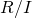and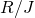. This sort of thing is usually left as an exercise (especially the first Corollary) and not proved in full generality in algebra courses, although it is not hard.

In all that follows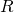is a commutative ring with identity and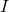and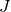are ideals of.

Lemma: If, there is a natural map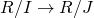.

Proposition: The natural map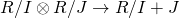is an isomorphism of-algebras.

Proof: To see surjectivity, notice thatgenerates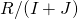as an-module, and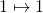, since the map is a ring homomorphism. To see injectivity, notice that every element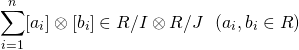is equal to the pure tensor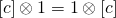where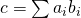. If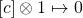, then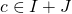. So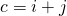,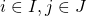. Then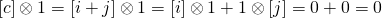.

Corollary: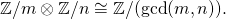Proposition (Chinese Remainder Theorem): The natural map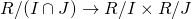is injective. If, it is also surjective, and thus an isomorphism.

Proof: To see injectivity, notice that if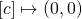, then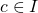and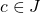, so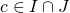so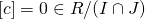. To see surjectivity, note thatimplies there exist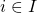,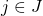, such that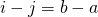, for any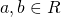. Consider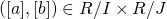, and setand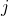. Then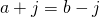, so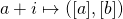.

Corollary: If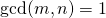,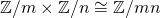. In particular, by applying this repeatedly we have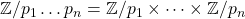.

Also, note the following fact:

Proposition: If, then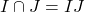.

Proof: Clearly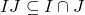. To go the other way, note thatmeans that there exist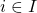,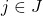, and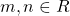such that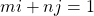. So, consider an element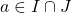. Then we have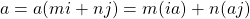. Since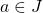,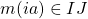, and since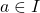,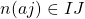. So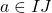.

Remark: One can also think of this in terms of Tor: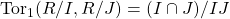, and when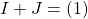this Tor group vanishes.